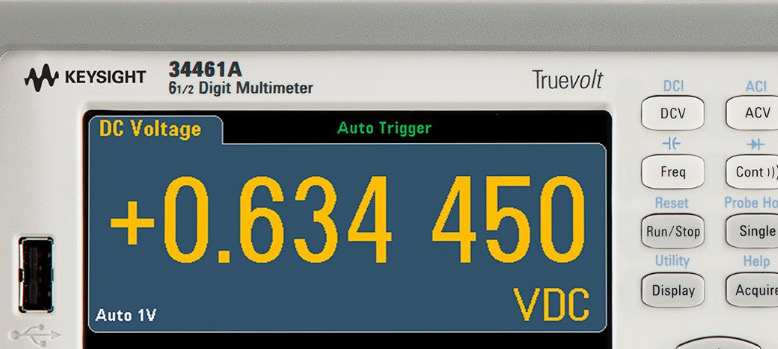# PrecisionWritten by

In the field of electronics, you often hear the word “precision” bandied around, but what does it actually mean? Sometimes precision is confused with accuracy, which is a related but different concept. What exactly is precision and why should you care?

Both precision and accuracy are ways of looking at the error associated with measurements of physical or electrical properties. Accuracy is a measure of how close the measurement is to a “true” value, or more likely in practice, how closely a value is relative to an accepted standard such as a metre, volt, or amp. Precision, on the other hand, is a measure of how close repeated measurements of the same quantity are to each other. This is a factor of the measurement set-up.

Figure 1 shows the difference between precision and accuracy in statistical terms. In this case the precision is shown by the spread distribution of measurements. I’ve shown this as a discrete distribution since most of the measurements we take in electronics are digitised somehow. The tighter the spread, the higher the precision. Accuracy is measured by the distance between the mean of measurements and the reference of “true” value.FIGURE 1. Precision describes the error associated with the repeatability of a measurement, shown here as the width of the distribution. Accuracy describes how close the measurement (or mean of several measurements) differs from a true or accepted value.

While the accuracy of a measurement is usually expressed as an error in percent or parts per million, and it is possible to express precision in that way too, it is very useful to think about precision in terms of significant figures. This is especially true if you are going to perform any kind of maths relating to real-world measured quantities.

Before we get into that, let’s look at an example of the misuse of precision. We often see spurious precision related to unit conversion. How many times have you seen something in print like “… the vehicle was estimated to be travelling at over 80.47 kilometres per hour…”? This seems awfully precise for a human observer. Of course, 80.47 kilometres per hour is an over precise conversion of 50 miles per hour. The original estimate probably has a precision of just one significant figure. The converted text should read “… the vehicle was seen travelling at over 80 kilometres per hour…”

This illustrates an important point about precision. We infer something about the precision of a measurement by the way it is written. For example. we would assume 169.3 has 4 significant figures, or else why include the decimal and the last digit? Similarly, we would assume 169.0 has four significant figures, since the decimal point and the trailing zero would not be necessary otherwise. The number 169 has three significant figures. But what about a number like 170? There is no way to tell if the last zero is significant or if it is just a placeholder. This could have either two or three significant figures.

To avoid this ambiguity, we could use scientific notation: 1.7 x 102 tells us unambiguously that there are 2 significant figures and 1.70 x 102 has 3 significant figures. We can also use units carefully to avoid implying spurious precision, for example using 12.3kV instead of 12,300V where we have only three significant figures.

We have to watch it when we are doing maths, as we have seen above. If I measure 2.3A at 1.65V in my circuit, the most I can say about the resulting power is that it is 3.8 Watts. This is because the current measurement only has two significant figures. If I were to write 3.795 Watts, which is what a calculator may suggest, I will have added 2 spurious significant figures. For multiplication and division, the significant figures of the result will only be as good as the input with the lowest precision.

For addition and subtraction, the rules are a bit different. You need to look at the significant digits in the fractional part of the number. The result cannot have a fractional part with more significant figures than that of the least precise input. For example, 990.2 + 11.234 should be expressed as 1001.4, not 1001.434. For addition and subtraction, the result cannot contain more decimal places than the lease precise input.

You always should keep the spurious precision for intermediate calculations to avoid cumulative rounding errors. Adjust your result at the end to restore proper precision.

You may ask why all this matters. I can assure you that is does—specifying more precision than required can cost a lot of money. If you need a current of 7mA with a voltage drop of 3.3V, there is a hell of a difference in cost between a 471.428Ω (0.01%) resistor and a 470Ω (5%) one. It’s even more important if you ever need to use a machine shop. It will cost you a whole lot more to have a dimension machined to 10.000mm than it will to 10.0mm.

Reference:
James, Dustin. “Significant Figure Rules”. PDF. http://www.ruf.rice.edu/~kekule/SignificantFigureRules1.pdf

 Keep up-to-date with our FREE Weekly Newsletter! Don't miss out on upcoming issues of Circuit Cellar. Subscribe to Circuit Cellar Magazine Note: We’ve made the May 2020 issue of Circuit Cellar available as a free sample issue. In it, you’ll find a rich variety of the kinds of articles and information that exemplify a typical issue of the current magazine. Would you like to write for Circuit Cellar? We are always accepting articles/posts from the technical community. Get in touch with us and let's discuss your ideas.